# Integral Resources

•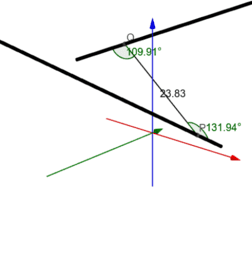### Distance between skew lines

Activity

Integral Resources

•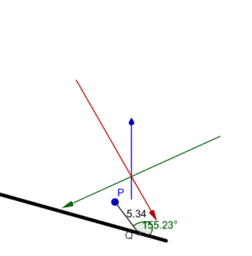### Distance from point to line

Activity

Integral Resources

•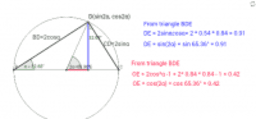### The double angle formulae

Activity

Integral Resources

•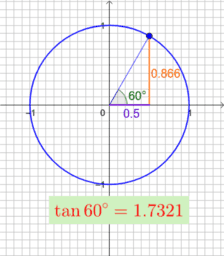### The graph of y = tan x

Activity

Integral Resources

•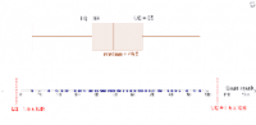### Boxplots and outliers

Activity

Integral Resources

•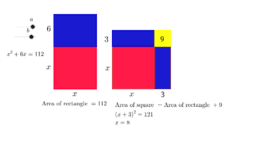### Completing the square and area

Activity

Integral Resources

•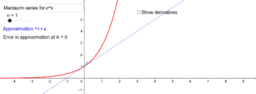### Maclaurin series approximations

Activity

Integral Resources

•### The graph of y = |f(x)|

Activity

Integral Resources

•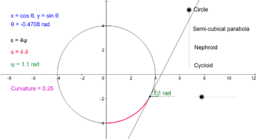### Intrinsic equations

Activity

Integral Resources

•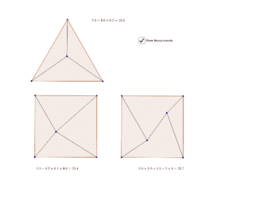### Minimum Connector for Corners of a Square

Activity

Integral Resources

•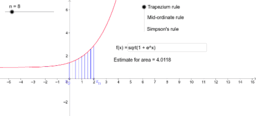### Numerical Integration

Activity

Integral Resources

•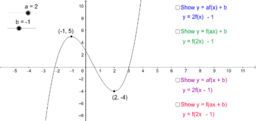### Combined transformations

Activity

Integral Resources

•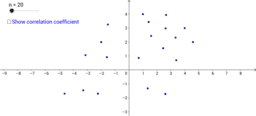### Correlation

Activity

Integral Resources

•### Collisions

Activity

Integral Resources

•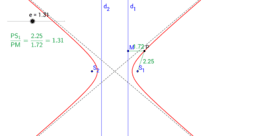### Conic sections

Activity

Integral Resources

•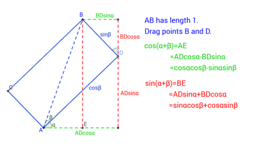### The compound angle formulae

Activity

Integral Resources